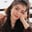Related Tags

iat
at
pandas
communitycreator
python

# What are the at() and iat() methods in pandas?Fouzia Bashir

### Overview of at[] method

Python provides many functions to help analyze data. The Python library pandas is particularly useful for data analysis. In pandas, the at[] function is used to access one value of a row and column in data. It is used with two parameters. The first is the position of the value and a column and the second is the title of the column.

Another pandas function, loc[], works similarly, but the at[] function is more efficient and faster because it can access one value at a time.

### Syntax

DataFrame.at[position_of_element, title_of_column]

### Parameters

• position_of_element: This is the position of the value and a column.
• title_of_column: This is the title of the column.

### Return value

Get the single value according to the title.

### Example

#Importing Pandas module
import pandas as pd

#Creating dataframe
data = pd.DataFrame([[0, 2, 4, 6, 8], [1, 3, 5, 7, 9], [5, 10, 15, 20, 25], [10, 20, 30, 40, 50]], columns=['First', 'Second', 'Third', 'Fourth', 'Fifth'])

#Creating position_of_value and title variables
position_of_value = 2
title = 'Second'

#Calling at[] method
result = data.at[position_of_value, title]

#Print the result
print(result)

Using the at[] method

### Explanation

• Line 2: We import the pandas module.
• Line 5: We create a DataFrame and assign it to data variable.
• Line 12: We initialize a variable named result to hold the value of .at[] method applied. The element position was given as the first parameter and title of a column as the second parameter.
• Line 15: We print the value of the result variable.

### Overview of iat[] method

The iat[] method is used to retrieve a pair value from row and column from data given in a DataFrame. Like the at[] method, it also has two parameters; the first is the position of the element in a row and the second is the position of the element in a column.

The iloc[] also works similarly to iat[] function, but it is faster because it works on a single value at a time.

### Syntax

DataFrame.iat[row_element,column_element]

### Parameters

• Row_element: This is the position of the element in a row.
• Column_element: This is the position of the element in a column.

### Return value

Get the single value of row and column.

#Importing Pandas module
import pandas as pd

#Creating Dataframe
data = pd.DataFrame([[0, 2, 4, 6, 8], [1, 3, 5, 7, 9], [5, 10, 15, 20, 25], [10, 20, 30, 40, 50]], columns=['First', 'Second', 'Third', 'Fourth', 'Fifth'])

#Creating column and row variables
col_value = 3
row_value = 2

#Calling .iat[] method
output = data.iat[row_value, col_value]

#Print the result
print(output)


Using the iat[] method

### Explanation

• Line 2: We import the pandas module.
• Line 5: We simply create a DataFrame and assign it to the data variable.
• Lines 8–9: We initialize two variables col_value and row_value to retrieve the value of the column and the row respectively. Next, we call the iat[] method to the values from the row and the column.
• Line 15: We print the result variable which contains the output.

RELATED TAGS

iat
at
pandas
communitycreator
python

CONTRIBUTORFouzia Bashir
RELATED COURSES

View all Courses

Keep Exploring

Learn in-demand tech skills in half the time